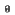The trigonometric method of vector resolution involves using trigonometric functions to determine the components of the vector. While this method is perhaps not as ‘entertaining’ as either of the others, it is (by far) the most accurate and easiest in the long run.

This method is based on the fact that any given vector can be separated into components that act at right angles to each other.

This method is by far (after you get used to it) the easiest and most reliable method for finding the resultant of any number of vector quantities.

Most textbooks use the cosine law for this method, but a quick, much easier method is to use the trig functions of each vector to separate it into x and y (vertical and horizontal) components, then combine the components.

Summary of equations:

x = r cosy = r sinx2 + y2 = r2= tan-1(y/x)EXAMPLE: the following forces act simultaneously on an object

V1 = 500 N force acts at an angle of 17o W of N

V2 = 315 N at 27o W of S

For the first vector:

Step1:   reference the angle back to due E
17o W of N = 90o + 17o from due E = 107o

Step 2:   now use the cos function to find the x (horizontal) component ( x = r cos)
cos 107o X 500 N = -146.186 N

Step 3:   use the sin function to find the y (vertical) component (y = r sin)
sin 107o X 500 N = 478.152 N

For the second vector:

Step1:    reference angle back to due E
27o W of S = 270o (due S) 27o (back west) from due E = 243o

Step 2:   use cos function to find x component
cos 243o X 315 N = -143.007 N

Step 3:   use sin function to find y component
sin 243o X 315 N = -280.667 N

• Notice that using this method, reference everything back to due E, means your calculator automatically gives you pos/neg, which is important when you combine the components!
• Now, combine the x components
-146.186 + (-143.007) = -289.193 N
• Combine the y components
478.152 + (-280.667) = 197.485 N
• Look at the signs of the resultant x and y: x is neg and y is pos, so your resultant will be in the 2nd quad; this means the angle of the resultant will be (using this method) N of due W
• Now use the Pythagorean Theorem to find the resultant (by separating the vectors into x and y components, you are forcing the resultant to be the hypotenuse of a right triangle).

Resultant2 = x2 + y2

= (-289.193)2 + (197.485)2

= sq rt (122632.9165)

resultant = 350.190 N

resultant = 350 N or 3.5×102 N

Now find the orientation (angle)

Tan angle (the angle of the resultant above due W) = y/x          [tan-1 (y/x)]
= 197.485/289.193

NOTE: you don’t need to use pos/neg here since you know its in the 2nd quad and both are negative

tan  -1 = 0.682883057
(use inv tan)
angle = 34o

So your resultant = 3.4 X 102 N 34o N of W

• Now go to Physics Classroom trig method on the following website and read through that explanation as reinforcement.   Use the Components of a Vector widget at the bottom of the page to resolve vectors into their components.

(source)